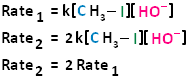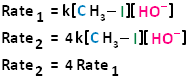# 11.2: The SN2 Reaction

$$\newcommand{\vecs}{\overset { \rightharpoonup} {\mathbf{#1}} }$$ $$\newcommand{\vecd}{\overset{-\!-\!\rightharpoonup}{\vphantom{a}\smash {#1}}}$$$$\newcommand{\id}{\mathrm{id}}$$ $$\newcommand{\Span}{\mathrm{span}}$$ $$\newcommand{\kernel}{\mathrm{null}\,}$$ $$\newcommand{\range}{\mathrm{range}\,}$$ $$\newcommand{\RealPart}{\mathrm{Re}}$$ $$\newcommand{\ImaginaryPart}{\mathrm{Im}}$$ $$\newcommand{\Argument}{\mathrm{Arg}}$$ $$\newcommand{\norm}{\| #1 \|}$$ $$\newcommand{\inner}{\langle #1, #2 \rangle}$$ $$\newcommand{\Span}{\mathrm{span}}$$ $$\newcommand{\id}{\mathrm{id}}$$ $$\newcommand{\Span}{\mathrm{span}}$$ $$\newcommand{\kernel}{\mathrm{null}\,}$$ $$\newcommand{\range}{\mathrm{range}\,}$$ $$\newcommand{\RealPart}{\mathrm{Re}}$$ $$\newcommand{\ImaginaryPart}{\mathrm{Im}}$$ $$\newcommand{\Argument}{\mathrm{Arg}}$$ $$\newcommand{\norm}{\| #1 \|}$$ $$\newcommand{\inner}{\langle #1, #2 \rangle}$$ $$\newcommand{\Span}{\mathrm{span}}$$

Objectives

After completing this section, you should be able to

1. write an expression relating reaction rate to the concentration of reagents for a second-order reaction.
2. determine the order of a chemical reaction from experimentally obtained rate data.
3. describe the essential features of the SN2 mechanism, and draw a generalized transition state for such a reaction.
##### Key Terms

Make certain that you can define, and use in context, the key terms below.

• bimolecular
• kinetics
• rate coefficient
• rate equation
• reaction rate
• second-order reaction
• SN2
##### Study Notes

Most of the key terms introduced in this section should already be familiar to you from your previous general chemistry course.

Reaction rate refers to the change in concentration of a reactant or product per unit of time. Using strict SI units, reaction rates are expressed in mol · L−1 · s−1, but in some textbooks you will find this value written as M/s. In general, the reaction rate of a given reaction changes with time, as it is dependent on the concentration of one or more of the reactants.

An equation which shows the relationship between the reaction rate and the concentrations of the reactants is known as the rate equation. All rate equations contain a proportionality constant, usually given the symbol k, which is known as the rate coefficient. Some textbooks refer to this value as the “rate constant,” but this name is a little misleading as it is not a true constant. The rate coefficient of a given reaction depends on such factors as temperature and the nature of the solvent.

SN2 is short for “bimolecular nucleophilic substitution.” You will encounter abbreviations for other types of reactions later in this chapter.

If you are unclear on the point about the inversion of configuration during an SN2 reaction, construct a molecular model of a chiral alkyl halide, the transition state formed when this substance reacts with a nucleophile in an SN2 process, and the product obtained from this reaction.

In many ways, the proton transfer process in a Brønsted-Lowry acid-base reaction can be thought of as simply a special kind of nucleophilic substitution reaction, one in which the electrophile is a hydrogen rather than a carbon.In both reaction types, we are looking at very similar players: an electron-rich species (the nucleophile/base) attacks an electron-poor species (the electrophile/proton), driving off the leaving group/conjugate base.   Instead of showing a specific nucleophile like hydroxide, we will simply refer to the nucleophilic reactant as 'Nu'. In a similar fashion, we will call the leaving group 'X'. We will see as we study actual reactions that leaving groups are sometimes negatively charged, sometimes neutral, and sometimes positively charged. We will also see some examples of nucleophiles that are negatively charged and some that are neutral. Therefore, in this general picture we will not include a charge designation on the 'X' or 'Nu' species. We will generalize the three other groups bonded on the electrophilic central carbon as R1, R2, and R3: these symbols could represent hydrogens as well as alkyl groups. Here, then, is the generalized picture of a concerted (single-step) nucleophilic substitution reaction.

## General SN2 Reaction## Example## The SN2 Mechanism

Bimolecular nucleophilic substitution (SN2) reactions are concerted, meaning they are a one step process. This means that the process whereby the nucleophile attacks and the leaving group leaves is simultaneous. Hence, the bond-making between the nucleophile and the electrophilic carbon occurs at the same time as the bond-breaking between the electrophilic carbon and the halogen. This is called an 'SN2' mechanism. In the term SN2, S stands for 'substitution', the subscript N stands for 'nucleophilic', and the number 2 refers to the fact that this is a bimolecular reaction: the overall rate depends on a step in which two separate molecules (the nucleophile and the electrophile) collide.   The mechanism starts when lone pair electrons from the nucleophile attacks the electrophilic carbon of the alkyl halide to form a C-Nu sigma bond.  Simultaneously, X-C bond is broken when the electrons are pushed onto the leaving group.  Overall during this mechanism, a set of lone pair electrons are transferred from the nucleophile to the leaving groups.If you look carefully at the progress of the SN2 reaction, you will realize something very important about the outcome. The nucleophile, being an electron-rich species, must attack the electrophilic carbon from the back side relative to the location of the leaving group. Approach from the front side simply doesn't work: the leaving group - which is also an electron-rich group - blocks the way.The result of this backside attack is that the stereochemical configuration at the central carbon inverts as the reaction proceeds. In a sense, the molecule is turned inside out. SN2 reactions that begin with the R enantiomer as the substrate will form the S enantiomer as the product. Those that begin with the S enantiomer as the substrate will form the R enantiomer as the product. This concept also applies to substrates that are cis and substrates that are trans. If the cis configuration is the substrate, the resulting product will be trans. Conversely, if the trans configuration is the substrate, the resulting product will be cis.

What this means is that SN2 reactions whether enzyme catalyzed or not, are inherently stereoselective: when the substitution takes place at a stereocenter, we can confidently predict the stereochemical configuration of the product. Below is an animation illustrating the principles we have just learned, showing the SN2 reaction between hydroxide ion and methyl iodide. Notice how backside attack by the hydroxide nucleophile results in inversion at the tetrahedral carbon electrophile.## Example## Bimolecular Nucleophilic Substitution Reactions and Kinetics

In the term SN2, (as previously stated) the number two stands for bimolecular, meaning there are two molecules involved in the rate determining step. The rate of bimolecular nucleophilic substitution reactions depends on the concentration of both the haloalkane and the nucleophile. To understand how the rate depends on the concentrations of both the haloalkane and the nucleophile, let us look at the following example. The hydroxide ion is the nucleophile and methyl iodide is the haloalkane.If we were to double the concentration of either the haloalkane or the nucleophile, we can see that the rate of the reaction would proceed twice as fast as the initial rate.If we were to double the concentration of both the haloalkane and the nucleophile, we can see that the rate of the reaction would proceed four times as fast as the initial rate.The bimolecular nucleophilic substitution reaction follows second-order kinetics; that is, the rate of the reaction depends on the concentration of two first-order reactants. In the case of bimolecular nucleophilic substitution, these two reactants are the haloalkane and the nucleophile. For further clarification on reaction kinetics, the following links may facilitate your understanding of rate laws, rate constants, and second-order kinetics:

Exercise $$\PageIndex{1}$$

1) The reaction below follows the SN2 mechanism.a) Write the rate law for this reaction.

b) Determine the value of the rate coefficient, k, if the initial concentrations are 0.01 M CH3Cl, 0.01 M NaOH, and the initial reaction rate is 6 x 10-10 M/s.

c) Calculate the initial reaction rate if the initial reactant concentrations are changed to 0.02 M CH3Cl and 0.0005 M NaOH.

2)  Predict the product of a nucleophilic substitution of (S)-2-bromopentane reacting with CH3CO2-, Show stereochemistry.

3) Predict the structure of the product in this SN2 reaction. Be sure to specify stereochemistry.4)  Since everything is relative in chemistry, one reaction's nucleophile can be another reaction's leaving group. Some functional groups can only react as a nucleophile or electrophile, while other functional groups can react as either a nucleophile or electrophile depending on the reaction conditions. Classify the following compounds as nucleophile, electrophile, or leaving groups. More than one answer may be possible.

a) bromoethane

b) hydroxide

c) water

d) chlorocyclohexane

e) ethanol

f) bromide

1)  a) rate = k [CH3Cl] [OH-]

b) substitute the data into the rate expression above and apply algebra to solve for k

k = 6 x 10-6 Lmol-1s-1

c) Using the rate law above, substitute the value for k from the previous question along with the new concentrations to determine the new initial rate.

rate = 6 x 10-10 M/s

$\begin{array}{r}2\right)\end{array}$3)4)

a) electrophile (Alkyl halides are always electrophiles - one reason they are an o-chem student's best friend.)

b) strong nucleophile

c) weak nucleophile and good leaving group

d) electrophile (Alkyl halides are always electrophiles - one reason they are an o-chem student's best friend.)

e) weak nucleophile, a poor electrophile without clever chemistry (stay tuned for future chapters), good leaving group

f) good nucleophile and a good leaving group